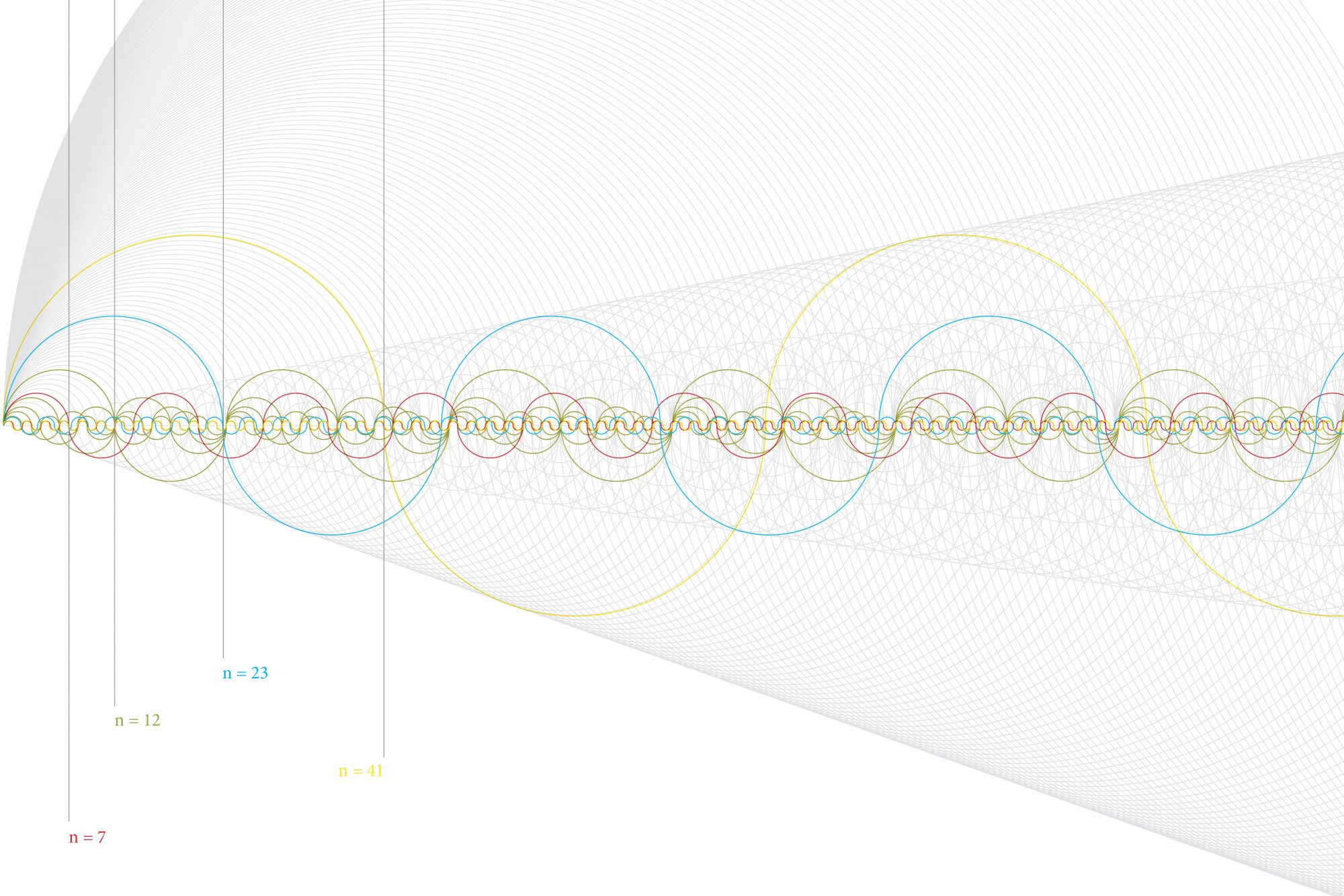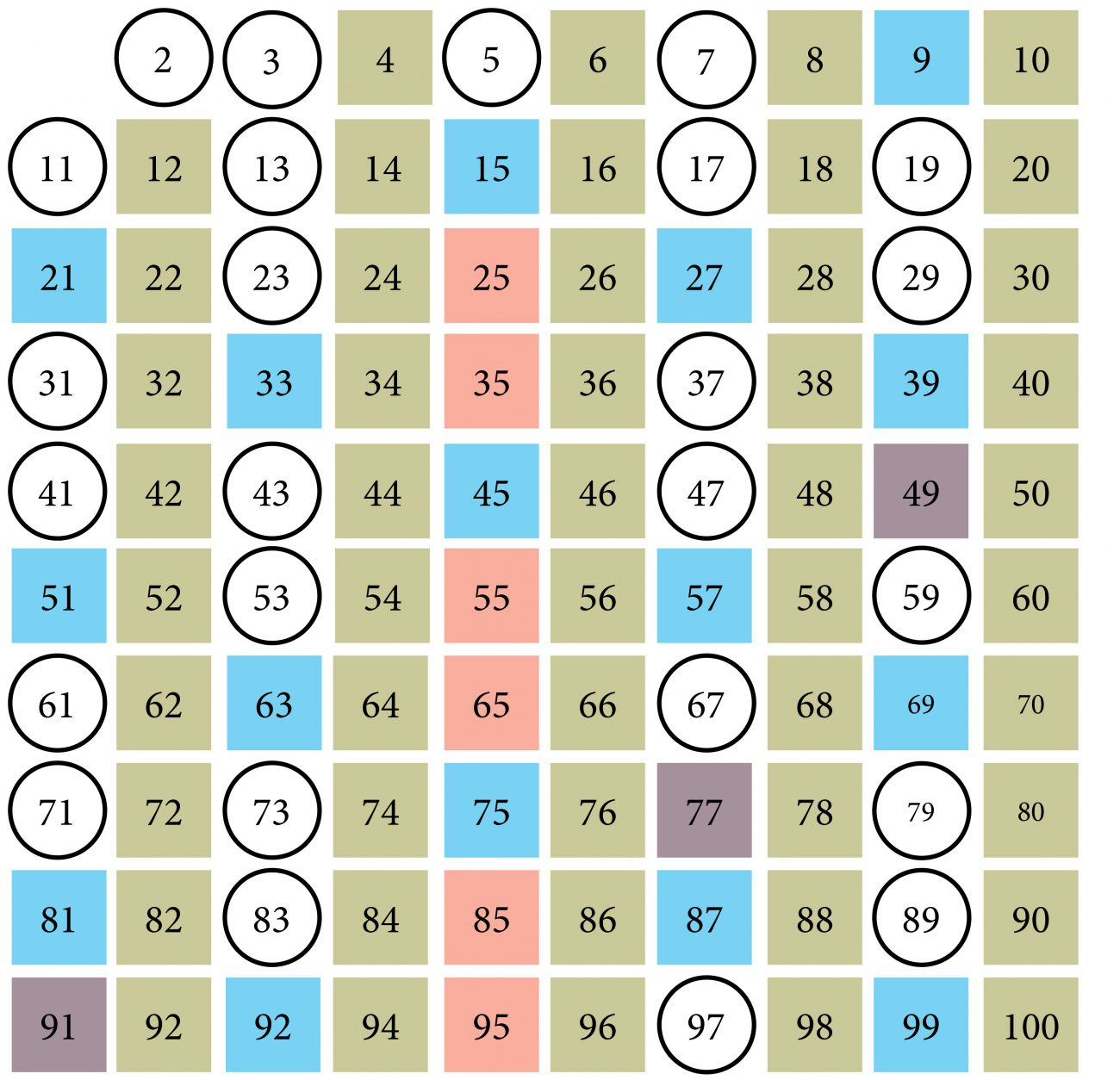## Pattern recognition

Prime numbers act as locks and keys to bank accounts—and cracking them requires solving a riddle that dates back to ancient times.

If you were paying attention in maths, you’ll know that a prime number can be divided only by itself, or one, without leaving a remainder. Primes are quite common, at least to begin with—2, 3, 5, 7, 11, 13, 17, 19 are prime numbers—but they become fewer and fewer as you go. Except for 2, even numbers can’t be primes, and the higher you count, the more likely an odd number is to be divisible by 3, or 5, or 7, meaning it isn’t a prime.

To be honest, this doesn’t excite me now any more than it did at school, but it turns out I should be more mindful of primes, and so should you, because they’re all that’s stopping some cyber-delinquent from emptying your bank account.

This is because prime numbers are the building blocks of mathematics. You can make any non-prime number you want using only primes—this is called a composite. (The number 6, for instance, is a composite, because you can create it by multiplying the prime numbers 2 and 3.)

Primes are organised a bit like the universe—close to home, they’re clumped together, but as you journey farther towards infinity, they become sparse. If you count from 9,999,000 to 10,000,000, you’ll find only 53 prime numbers, for example.

But, like the universe, primes occasionally and inexplicably go into huddles: right after 100, for instance, there’s a bunch of them—101, 103, 107, 109. It’s that elastic distribution that continues to confound mathematicians.

Why does any of this matter? Because the weird properties of primes lend them perfectly to modern life. Every time you use your ATM, it’s prime numbers that protect your account.

One of the most common encryption schemes, the RSA algorithm, creates your ‘public key’ by multiplying two very large prime numbers, and decrypts it with a ‘private key’, which is those two primes themselves.

While I can quickly figure out that the factors of 20 are 2 x 2 x 5, I don’t have enough years left to figure out that 2,244,354 is 2 x 3 x 7 x 53,437. Determining the prime factors of a very large number is a Herculean task: it took researchers two years and hundreds of parallel computers to identify the factors of a 232-digit number, so you can sleep easy knowing that the primes offer impenetrable shelter for your data, and those of international commerce.This diagram shows the factors of four numbers. The composite number 12 is intersected by numerous curves representing its factors—curves representing 1, 2, 3, 4, 6 and 12. Prime numbers have only two curves, representing 1 and the number itself.

But they have another vexing trait that’s been baffling the best brains for more than two millennia. Given that primes get rarer as you go, shouldn’t they eventually disappear completely?

Euclid of Alexandria was brainstorming this back in 300 BC. If there is only a finite number of primes, he postulated, then there must be a last prime, after which everything up to infinity is, instead, a composite number.

To test the problem, he imagined multiplying a set of prime numbers, then adding 1. Next, he pondered the two possible outcomes. Is the resulting number, call it n, a prime or not? If it isn’t—if it’s a composite—it must have a prime factor, which Euclid demonstrated could not have been one of the set of prime numbers.

For instance, n = (2 × 7 × 11) + 1 = 155. That’s a composite number, but its prime factors—5 and 31—are not among the prime numbers multiplied together. If, on the other hand, n is a prime, it must be greater than the primes used in the calculation. In either event, Euclid proved that primes are, against all odds, infinite.

He also believed there is an infinite number of prime pairs. You may have noticed that our primes so far have hung out together—3 and 5, 17 and 19, 101 and 103. In each case, the difference is 2. So, are prime pairs infinite?

In 1846, French mathematician Alphonse de Polignac found that any even number can be expressed in infinite ways, as the difference between two consecutive primes. Two, for instance, can be the product of 5 minus 3, or 7 minus 5, or 13 minus 11, and so on. No matter where they fall in the number line, de Polignac’s twin prime conjecture predicts there will always be a greater pair somewhere ahead. Proving him right, however, has been a tough gig.The sieve of Eratosthenes offers a simple algorithm for finding prime numbers. First, cross out 1, which isn’t a prime, then cross out the multiples of 2 and 3. Next, cross out the multiples of any number not yet crossed out—for instance, the multiples of 5 and 7. Finally, circle the numbers that remain. These are primes.

In 2005, Daniel Goldston of San José State University in California, János Pintz of the Alfréd Rényi Institute of Mathematics in Budapest and Cem Yıldırım of Boğaziçi University in Istanbul showed that there will always be pairs of primes occuring closer together than is predicted by the average spacing of primes. That doesn’t mean prime pairs are infinite, but it offered a methodology that Yitang (Tom) Zhang of the University of New Hampshire used in 2013 to calculate there are infinitely many pairs of primes that differ by some number, call it z, which is smaller than 70 million. Zhang’s bounded gap conjecture proved that the gap between prime pairs doesn’t necessarily widen out to infinity.

That’s still a long way from de Polignac’s twin prime conjecture (in order to prove that, someone will need to show that Zhang’s bounded gap conjecture is true for z = 2) but supercomputers may well narrow the gap. As of June 2018, the largest known prime number had 23,249,425 digits.

That complexity should keep your PIN safe—until the crooks get quantum computers. By imitating the schizophrenic properties of quantum particles, future computers will be able to process colossal volumes of information, using processors more than a million times faster than the one in your laptop. Quantum computers are for now a hypothesis, but one day they’ll storm that portal into the infinite, and hunt down prime pairs in the distant reaches of a maths galaxy that Euclid could barely conceive.

And you’ll need to come up with a better password.

More by